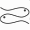# An Introduction to Functions

Suppose you are interested in understanding the temperature deviations in your house. The temperature may be high near the kitchen and low in a room that doesn't get much sun. In the winter time, the temperature near radiators, vents, or baseboards may be substantially warmer than the temperature away from sources of heat.

To measure the temperature in your house, you might place a thermometer at a variety of positions, and record the temperature. Although you would not know the temperature at every point in the house (did you crawl under the sink?), you could probably get a good idea of how the temperature varies from room to room.

One of the roles of science and mathematics is to model and predict natural phenomenon. A scientist will use knowledge of your house (e.g., the location of heat sources, the amount of insulation in your walls and attic, the temperature outside) to build a mathematical model of the heat distribution in your house. We say that the model is a "good model" if it can predict actual temperature measurements.

Scientists and engineers use functions to model phenomenon such as temperature. To each point in your house, we can associate a temperature. Thus a function is a process that takes an input (e.g., a point in the room) to an output (e.g., the temperature at that point). We call the set of inputs to a function the domain of that function. The set of all outputs we call the range of the function. In our example, the domain of the temperature function is a set of points, the range of our function is the set of temperatures reached by some point in your house. Sometime visualizing functions becomes easier if we ask the question: for a given value of the output, what is the subset of the domain that are associated to that value? In our temperature example, this is the question "what is the set of points in the house which are exactly 22 degrees Celsius (72 degrees Fahrenheit)?" The set of points which satisfy this condition are called an isotherm. (We see isotherms daily on weather maps.) We say that the isotherm is defined implicitly since it is the set of points that satisfies a condition.

Scientists can learn a lot by analyzing functions. For example, if your oven is on, the temperature in your kitchen changes rapidly over a short distance between the refrigerator and the center of the oven. Mathematicians quantify this behavior by saying that the temperature function is changing rapidly. The change in a function can be measured by looking at the so-called derivative function: When the temperature is not changing we say it has zero derivative; when the temperature is changing rapidly, we say that it has a large derivative.

Next: Level Sets of Single-Variable FunctionsThe Pisces Home Page